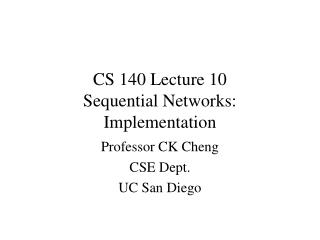Download PresentationCS 140 Lecture 10 Sequential Networks: ImplementationCS 140 Lecture 10 Sequential Networks: Implementation - PowerPoint PPT Presentation

Download PresentationCS 140 Lecture 10 Sequential Networks: Implementation
An Image/Link below is provided (as is) to download presentation

Download Policy: Content on the Website is provided to you AS IS for your information and personal use and may not be sold / licensed / shared on other websites without getting consent from its author. While downloading, if for some reason you are not able to download a presentation, the publisher may have deleted the file from their server.

- - - - - - - - - - - - - - - - - - - - - - - - - - - E N D - - - - - - - - - - - - - - - - - - - - - - - - - - -
Presentation Transcript

1. CS 140 Lecture 10Sequential Networks: Implementation Professor CK Cheng CSE Dept. UC San Diego

2. Implementation • Format and Tool • Procedure • Excitation Tables • Example

3. Canonical Form: Mealy and Moore Machines x(t) y(t) Combinational Logic CLK x(t) C2 y(t) x(t) C1 C2 y(t) C1 CLK CLK

4. Canonical Form: Mealy and Moore Machines Moore Machine: yi(t) = fi(X(t), S(t)) Mealy Machine: yi(t) = fi(S(t)) si(t+1) = gi(X(t), S(t)) x(t) x(t) C1 C2 y(t) C1 C2 y(t) CLK CLK s(t) s(t) Moore Machine Mealy Machine

5. Sequential Network Implementation:Format and Tool Canonical Form: Mealy & Moore machines State Table  Netlist Tool: Excitation Table x(t) C1 C2 y(t) CLK s(t) D(t) = h(x(t), S(t)) y(t) = f(x(t), S(t))

6. x PS Input Q(t) NS, y Implementation: Procedure Given a state table • State Table => Excitation Table we have NS = Q(t+1) = h(x(t),Q(t)) Output y(t) = f(x(t),Q(t)). We want to express D(t), T(t), S(t), R(t), J(t), K(t) as a funciton of inputs X(t) and current state Q(t). We derive the implementation of D, T, S, R, J, K as combinational logic.

7. Implementation: Procedure • State Table: y(t) = f(Q(t), x(t)) Q(t+1) = h(x(t),Q(t)) • Excitation Table: • D(t) = eD(Q(t+1), Q(t)); • T(t) = eT(Q(t+1), Q(t)); • S, R, J, K • From 1 & 2, we derive • D(t) = gD(Q(t), x(t)); • T = gT(Q(t), x(t)); • S,R,J,K. • Use K-Map to derive optional combinational logic implementation. • T(t) = gT(Q(t), x(t)) • y(t) = f(Q(t), x(t))

8. JK 00 0 1 11 1 0 10 1 1 01 0 0 0 1 Q(t+1) Q(t) Q(t+1) NS PS 0 0- -1 1 1- -0 0 1 Q(t) JK Excitation Table State table of a JK flip flop: Excitation table for a JK F-F: If Q(t) is 1, and Q(t+1) is 0, then JK needs to be 0-.

9. Excitation Tables and State Tables Excitation Tables: State Tables: SR SR Q(t+1) NS SR PS PS 0 0- 01 1 10 -0 00 0 1 01 0 0 10 1 1 11 - - 0 1 0 1 Q(t) Q(t) Q(t+1) T T Q(t+1) NS T PS PS 0 0 1 1 1 0 0 0 1 1 1 0 0 1 0 1 Q(t) Q(t) Q(t+1)

10. Excitation Tables and State Tables Excitation Tables: State Tables: JK JK Q(t+1) NS JK PS PS 0 0- -1 1 1- -0 00 0 1 01 0 0 10 1 1 11 1 0 0 1 0 1 Q(t) Q(t) Q(t+1) D D Q(t+1) NS D PS PS 0 0 0 1 1 1 0 0 0 1 1 1 0 1 0 1 Q(t) Q(t) Q(t+1)

11. J Q Q’ K C1 T Implementation: ExampleImplement a JK F-F with a T F-F Q(t+1) = h(J(t),K(t),Q(t)) = J(t)Q(t)+K(t)Q(t) State Table JK JK PS 00 0 1 01 0 0 10 1 1 11 1 0 0 1 Q(t)

12. Example: Implement a JK flip-flip using a T flip-flop Excitation Table of T flip-Flop T(t) = Q(t) XOR Q(t+1) Q(t+1) NS PS 0 0 1 1 1 0 0 1 Q(t) T Excitation Table of the Design id 0 1 2 3 4 5 6 7 J(t) 0 0 0 0 1 1 1 1 K(t) 0 0 1 1 0 0 1 1 Q(t) 0 1 0 1 0 1 0 1 Q(t+1) 0 1 0 0 1 1 1 0 T(t) 0 0 0 1 1 0 1 1 T(t) = Q(t) XOR ( J(t)Q’(t) + K’(t)Q(t))

13. Example: Implement a JK flip-flip using a T flip-flop T(J,K,Q): K 0 2 6 4 0 0 1 1 T = K(t)Q(t) + J(t)Q’(t) 1 3 7 5 Q(t) 0 1 1 0 J J Q Q’ T K